Recently, when I was helping a client with EMI issues on their automotive product, I bought an off-the-shelf filter from a well-known manufacturer. This was a quick-fix and was implemented as a proof of concept. After proving that the filter was effective, the next job was to design our own filter so we can fit the filter in the product with a reasonable size and cost. Naturally, the question came, how effective is our own filter compared with this off-the-shelf part?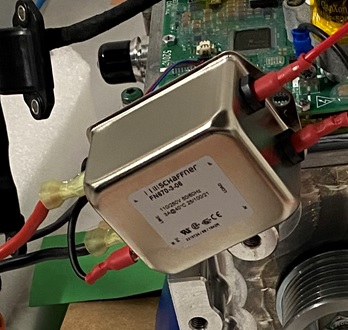Figure 1 An off-the-shelf filter from Schaffner used in troubleshooting stage

A quick simulation can give design engineers a good confidence level when designing a customized filter solution. However, a simulation model needs to be accurate enough so that the result does not over-predict or under-predict the filter performance (which is usually characterized as insertion loss). Unfortunately, when it comes to EMC, due to the prevalence of parasitic parameters and the nonlinearity associated with frequency, simulation modeling often takes a long time to develop and may not predict a result that agrees with the experiment.

In this case, we have the datasheet from the manufacturer that provides a reasonable result. However, we can use this filter as a benchmark and can aim for a simulation that would agree with experimental results. Once the simulation model is proved to be accurate enough, we can then quickly design our own filter and gain a good confidence level with an implemented design of our choosing. This article summarizes our approach to build a sufficiently accurate filter simulation, step by step.

### Understanding filter performance measurement per CISPR 17

According to , CISPR 17 defines the measurement set-ups for filters. It is understood that CISPR 17 provides a measured impedance that provides an optimal filter performance prediction (in reality the impedance of neither the source nor the load is 50Ω). As a result, manufacturers sometimes provide insertion loss results in four representative topologies, as shown in Figure 2.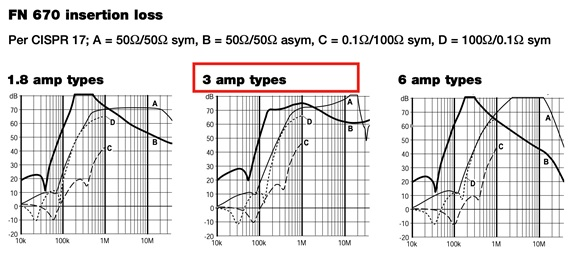Figure 2 Insertion loss shown in the datasheet, four set-ups were tested, mode A-D

### A simplified simulation model in SPICE simulation tool

A SPICE-based simulation tool provides a quick and cost-effective way of filter performance analysis. In our particular situation, a filter is modeled using SIMetrix (the model can be easily built using other simulation tools such as LTSPICE). From the manufacturer’s datasheet, we know the off-the-shelf filter is a multi-stage filter shown in Figure 3.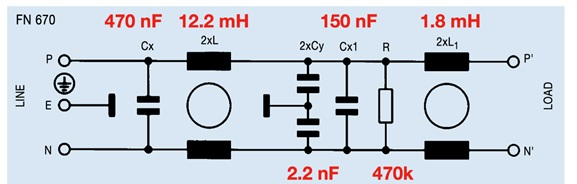Figure 3 Electric schematics of the off-the-shelf filter

A simplified simulation model is built as shown in Figure 4. Symmetrical (differential mode) performance can be analyzed by using AC analysis. To wit, Rsource and Rload have three combinations of impedances: 50Ω/50Ω (mode A), 0.1Ω/100Ω(mode C) and100Ω/0.1Ω(mode D). Figure 5 demonstrates the asymmetrical (common mode) 50Ω/50Ω (mode B) simulation.

The AC analysis sweeps between 10 kHz and 30 MHz for mode A and B. For mode C and D, the AC analysis is swept between 10 kHz and 1 MHz, same as the datasheet. This is because when the frequency goes above 1 MHz, asymmetrical mode (common mode) starts dominating.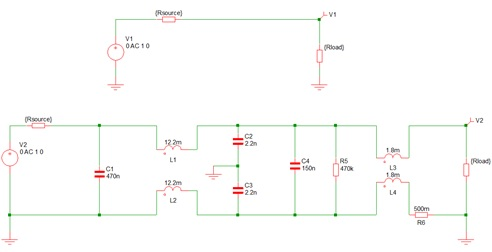Figure 4 Simplified filter model for symmetrical (differential mode) analysis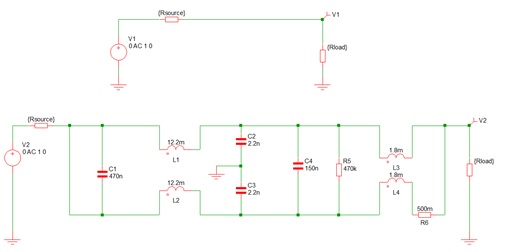Figure 5 Simplified filter model for asymmetrical (common mode) analysis

The common-mode choke (CMC) is modeled by defining a coupling factor between the two inductors in the simulation. To start with, a reasonable value of coupling is set as 0.99. When adjusting the coupling factor, the leakage inductance of the CMC changes. As one will soon find out, the leakage inductance of the CMC resonates with the capacitor Cx (C1 in the simulation file), it determines the first order response of the filter performance.

Running a quick simulation and plotting the dB relative voltage  gives the results shown in Figure 6. In comparison, the test results in the datasheet are presented on the left-hand side. One can see that below 200 kHz, the simulation results are quite close to the measurement results. However, as the frequency increases, parasitic components in the actual filter begin to take effect. As a result, the simplified filter model—in Figure 5—simply cannot predict the filter performance above 200 kHz.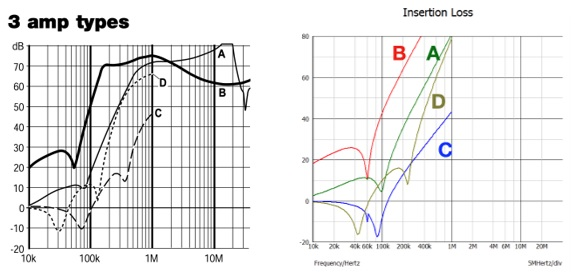Figure 6 Simulation results compared with experimental results in the datasheet

If you look at the comparison results shown in Figure 6, you can see 4 curves, A,B C and D represent four different test conditions, see Figure 2 in the article. So the simulation simulates four conditions, with A, C and D simulating the differential mode (as the model in Figure 4) and B simulates the common mode (as the model shown in Figure 5). You can find more about this here.

Parasitic parameters of the capacitors and inductors (notably, the CMCs) are then added to the simplified simulation model. Multiple rounds of tuning lead to the final simulation model (Figure 7). The parasitic parameters of each passive component are estimated and tuned in the following steps:

1. Initial parameters are estimated. Experienced engineers will know that at high frequencies, a capacitor’s equivalent series inductor (ESL) starts taking effect (correlated with an inductor’s interwinding capacitance). Depending on the capacitor type and the connection to the PCB, the ESL and equivalent series resistance (ESR) values can be estimated.
2. Damping of the capacitor and inductors are important; damping resistors are necessary to minimize resonance-induced overshoot.
3. Once the insertion loss profile is formed, one can then tune the inductance and capacitance value to match the resonance(s).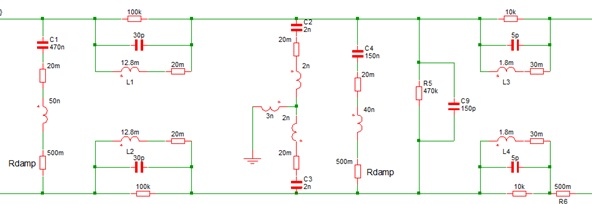Figure 7 Parasitic parameters are now introduced in the filter model

In any case, the simulation model is difficult to build, simply because one does not know the behavior of the passive components that are used in the off-the-shelf filter, In addition, we do not have the knowledge of how these components are connected inside the off-the-shelf filter.  Of course, one can take the extra steps of disassembling the filter and finding the details of each passive component. But for a quick job, the parasitic parameters can often be estimated based on basic knowledge of passive components and experience. When we design our own filters, we can often find detailed information of the parasitic parameters. This information is often presented in the form of resonance frequency (fres), we then can model the filter more accurately with this information.

It should be noted that the ESL of a capacitor is often overlooked by design engineers while the connections of the passive components are often ignored. Connections often introduce far more inductance than the internal ESL of a capacitor . In the simulation model shown in Figure 7, the ESL includes the inductance of the connections.

A 470nF X type film capacitor often has about 20 mΩ ESR with a resonant frequency that sits between 1 and 10 MHz . An estimated ESL of 20 nH generally goes well with this type of capacitor; another 30 nH inductance introduced by the connection is also taken into account. (So the lumped inductance value is shown as 50 nH.) Often, capacitors need to be damped to avoid oscillation (caused by LC tank resonance with the inductive component in the filter). The damping resistors are generally not shown in simplified schematics. In this case, a 500 mΩ damping resistor is put in series with the capacitor (shown as Rdamps in the simulation). The impact of a damping resistor can be seen in Figure 8.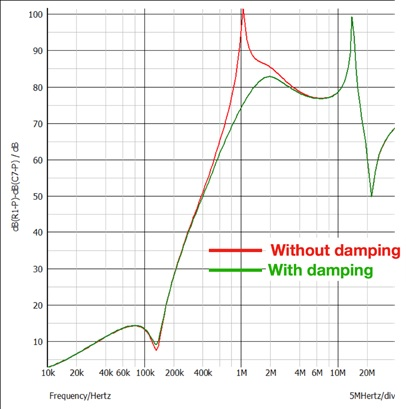Figure 8 Impact of damping resistor on capacitor

The coupling factor of both CMCs was tuned to be 0.999 to give a very strong common mode coupling. This leads to a leakage inductance of around 20-30 μH for the first stage CMC. Note that the leakage inductance depends on how the CMC is wound (sectional or bifilar), number of turns, and so on .

In this case, the leakage inductance is quite important for the differential mode filtering performance, particularly in the frequency range of a few MHz. Together with the capacitor C1, it also determines the poles of the filter transfer function. Another important parasitic parameter of the chokes is the interwinding capacitance, which depends on the number of turns and the distance between each winding. The values for CMCs are tuned to be 30pF and 5pF to match the resonance point.

It should be noted that the interwinding capacitance values seem to be extremely low in this case, as we were expecting an order of 100 pF for a 12.8 mH CMC. This is due to the over simplicity of the CMC model we used. In reality, the common mode and the leakage inductance reduce with frequency and current. Therefore, the same resonant frequency in the insertion loss (measured) means the interwinding capacitance is much larger than that of the simple simulation model.

While the profile of the 50Ω/50Ω filter setup simulation matches the datasheet measurement well, there’s a 10 dB over prediction of the simulation model between the frequency range of 1 and 10 MHz (see Figure 9). It is not clear what caused the difference, but we assume this is due to the frequency dependency of the leakage inductance of the CMC. Both high frequency eddy current and the proximity effect between adjacent layers could lead to reduction of leakage inductance. In fact, this drop could be significant, as it was found in , where the leakage inductance of a transformer dropped from 200 nH to 140 nH between 100 kHz and 1 MHz.

### Final simulation results

The simulation model in Figure 7 gives the filter performance results shown in Figure 9. Again, the datasheet results are shown as a comparison. The simulation results now match the test results much more accurately.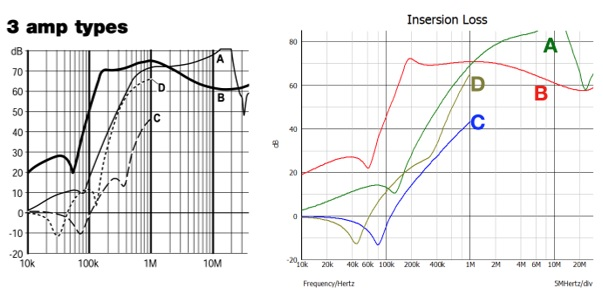Figure 9 Final simulation results compared with the results in the datasheet

### Application and areas of improvement

Once the simulation model is validated such as the case demonstrated above, we gain a good confidence level of the simulation model and the approach we take. We can then evaluate our own designed filter in a similar simulation model. The best part of this approach is that designers avoid using complicated math equations and the parasitic parameters are considered. This is particularly useful for high frequency (200 kHz – 30 MHz) analysis.

There are areas of improvement for this quick simulation model. For instance, as one can see, there wasn’t a dip in the mode D filter result (compared with mode D in test results). This is most likely to do with the coupling in the common-mode chokes. The over prediction of the simulated filter performance and the small interwinding capacitance value suggests a frequency dependent inductor/common-mode choke model should be used. Another improvement one can make based on this model is to include saturation of the inductance as saturation of a magnetic part is often one of the main reasons that a filter fails to perform properly.

### Bibliography

  Schaffner, "CISPR 17 Measurements 50Ω / 50Ω versus 0.1Ω / 100Ω," [Online].  M. Zhang, "Insertion Loss and Filter Performance," Mach One Design, 2021. [Online].  T. Hobbing, EMC Question of the Week 2017-2020.  TDK, "Film Capacitors General technical information," [Online].  W. Electronik, Trilogy of Magnetics 5th extended and revised edition, 2019.  Ziwei Ouyang,Jun Zhang,William Gerard Hurley, "Calculation of Leakage Inductance for High Frequency Transformers," IEEE Transactions on Power Electronics, vol. 30, no. 10, pp. 5769-5775, 2015.